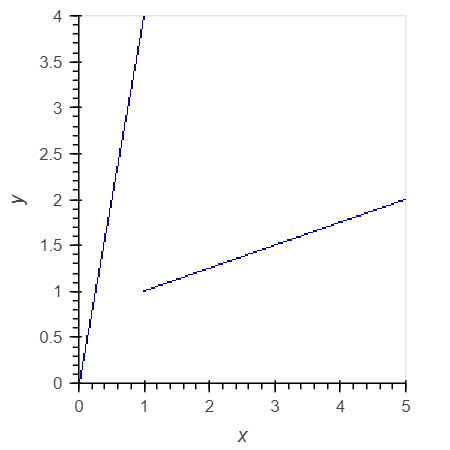# Drawing Bokeh Curve from single data frame row

Hi,
I am using Datashader and Bokeh to render a series of lines.
These are organised by row with the key columns being [x1, y1, x2, y2]
Is it possible to pass these to holoviews for interactive plotting?
I have managed to get it working by preprocessing to have in the HvCurve format where each entry is
[x1, y1]
[x2, y2]
[nan, nan]

But this is less efficient in terms of memory usage and requires pre-processing which impacts filtering.

If I try

``````            agg = HvCurve(ddf2, ('x1', 'x2'), ('y1', 'y2'))
``````

Then I get a lot of spurious extra lines.

Essentially, I can render images using:

``````from datashader.utils import export_image

cvs = ds.Canvas(plot_width=  4*800,
plot_height= 4*600,
)

imbuf = cvs.line(ddf2,
x=('x1', 'x2'),
y=('y1', 'y2'),
axis=1,
agg=ds.count('population'),
antialias=True
)

filename = "plot.png"
export_image(np, filename, background="black")
``````

can I do similar using Holoviews, with one row describing both points of the line?

Hello @sk2 , check out the `Segments` element.

``````import pandas as pd
import holoviews as hv